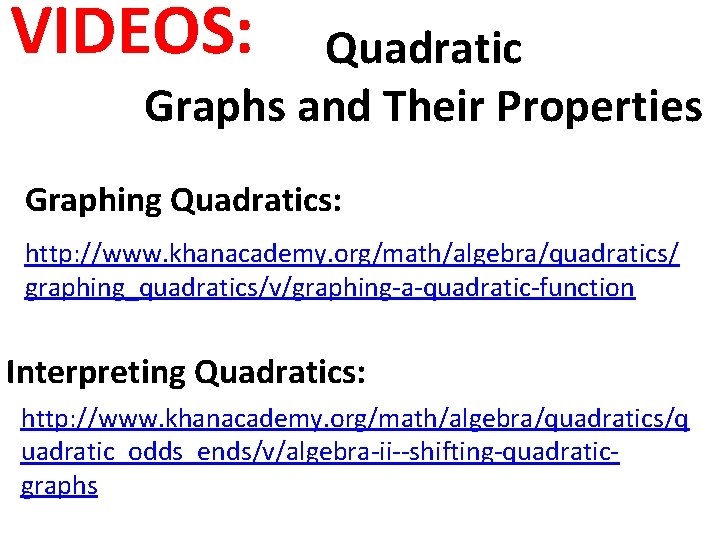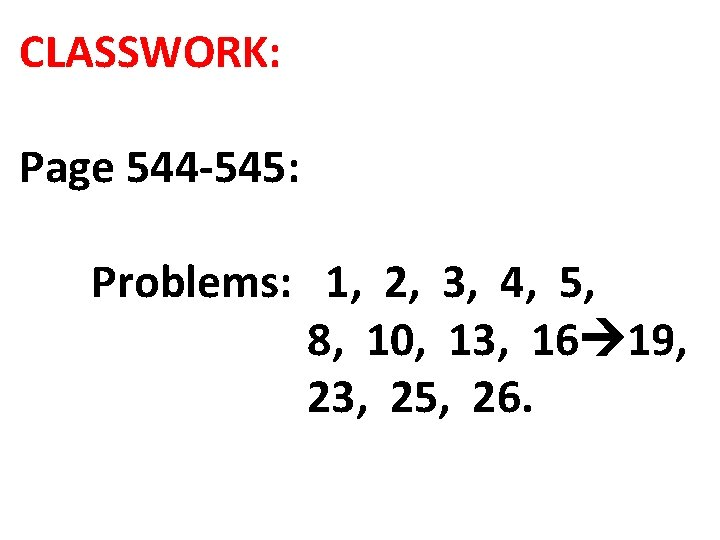• Slides: 19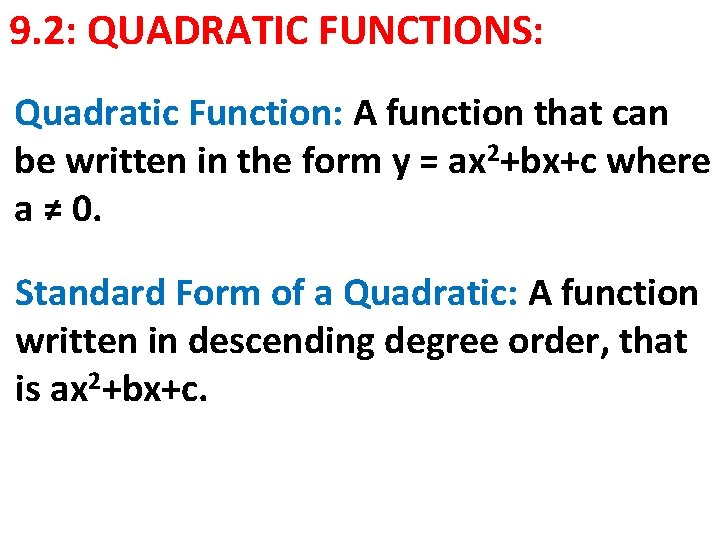9. 2: QUADRATIC FUNCTIONS: Quadratic Function: A function that can be written in the form y = ax 2+bx+c where a ≠ 0. Standard Form of a Quadratic: A function written in descending degree order, that is ax 2+bx+c.Quadratic Parent Graph: The simplest quadratic function f(x) = x 2. Parabola: The graph of the function f(x) = x 2. Axis of Symmetry: The line that divide the parabola into two identical halves Vertex: The highest or lowest point of the parabola.Minimum: The lowest point of the parabola. Maximum: The highest point of the Line of Symmetry parabola. Vertex = Minimum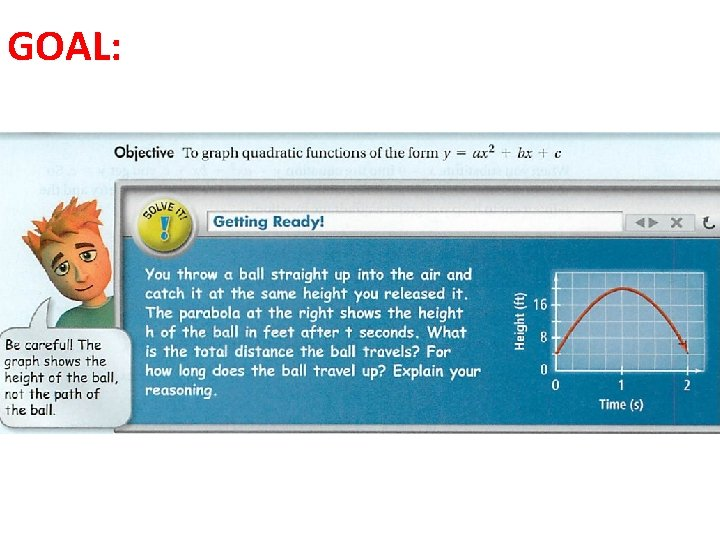GOAL:FINDING THE VERTEX OF 2 ax +bx+c: To find the vertex of a quadratic equation where a ≠ 1, we must know the following:Ex: Provide the graph, vertex, domain and range of: y= 2 3 x -9 x+2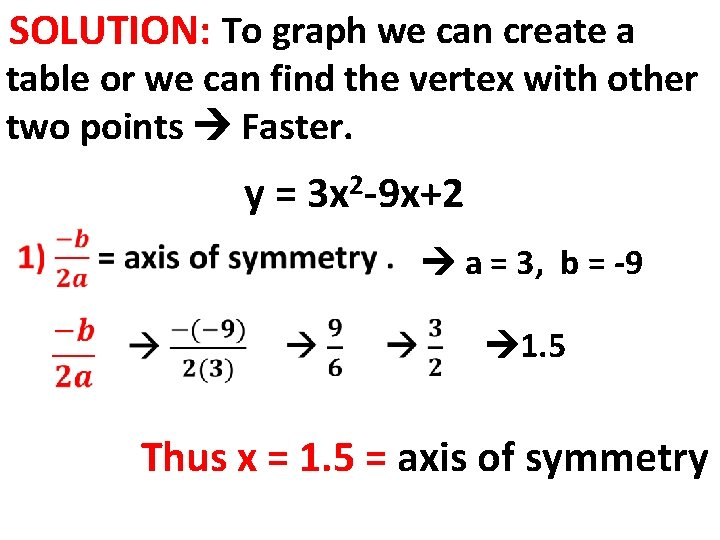SOLUTION: To graph we can create a table or we can find the vertex with other two points Faster. y = 3 x 2 -9 x+2 a = 3, b = -9 1. 5 Thus x = 1. 5 = axis of symmetry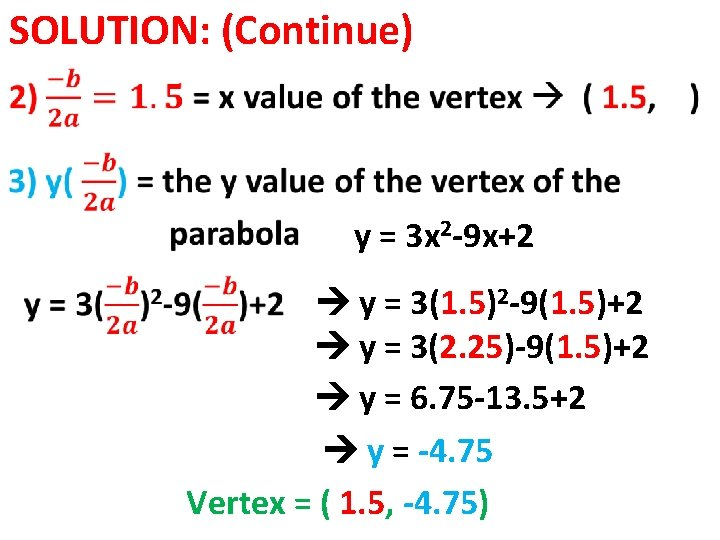SOLUTION: (Continue) y = 3 x 2 -9 x+2 y = 3(1. 5)2 -9(1. 5)+2 y = 3(2. 25)-9(1. 5)+2 y = 6. 75 -13. 5+2 y = -4. 75 Vertex = ( 1. 5, -4. 75)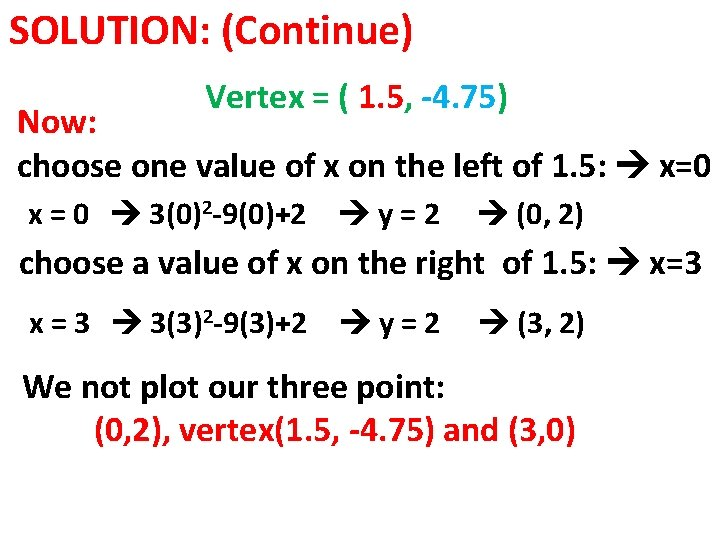SOLUTION: (Continue) Vertex = ( 1. 5, -4. 75) Now: choose one value of x on the left of 1. 5: x=0 x = 0 3(0)2 -9(0)+2 y=2 (0, 2) choose a value of x on the right of 1. 5: x=3 x = 3 3(3)2 -9(3)+2 y=2 (3, 2) We not plot our three point: (0, 2), vertex(1. 5, -4. 75) and (3, 0)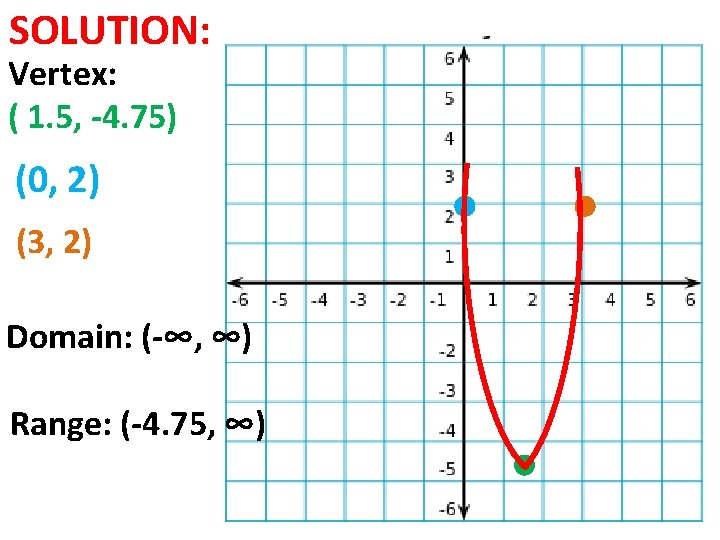SOLUTION: Vertex: ( 1. 5, -4. 75) (0, 2) (3, 2) Domain: (-∞, ∞) Range: (-4. 75, ∞)REAL-WORLD: During a basketball game halftime, the heat uses a sling shot to launch T-shirts at the crowd. The T-shirt is launched with an initial velocity of 72 ft/sec. The T-shirt is caught 35 ft above the court. How long will it take the T-shirt to reach its maximum height? What is the range of the function that models the height of the T-shirt over time?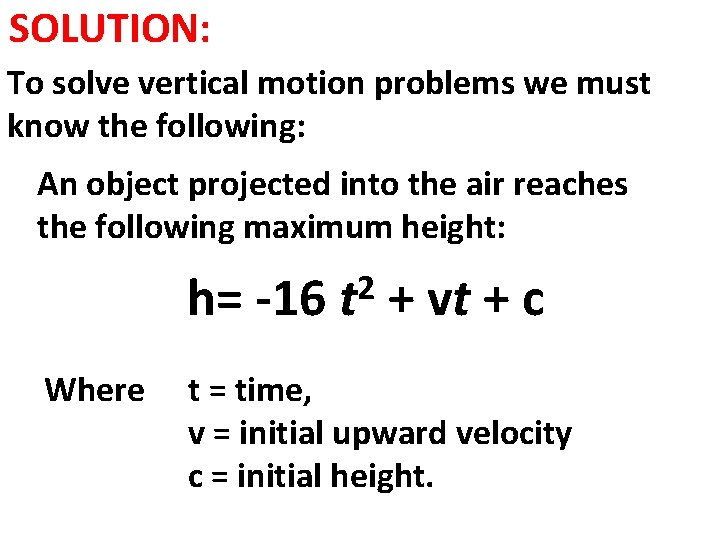SOLUTION: To solve vertical motion problems we must know the following: An object projected into the air reaches the following maximum height: h= -16 Where 2 t + vt + c t = time, v = initial upward velocity c = initial height.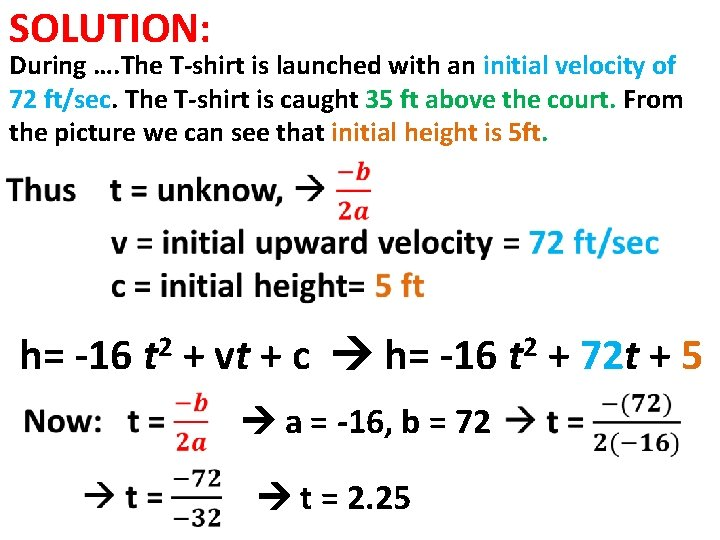SOLUTION: During …. The T-shirt is launched with an initial velocity of 72 ft/sec. The T-shirt is caught 35 ft above the court. From the picture we can see that initial height is 5 ft. h= -16 2 t + vt + c h= -16 a = -16, b = 72 t = 2. 25 2 t + 72 t + 5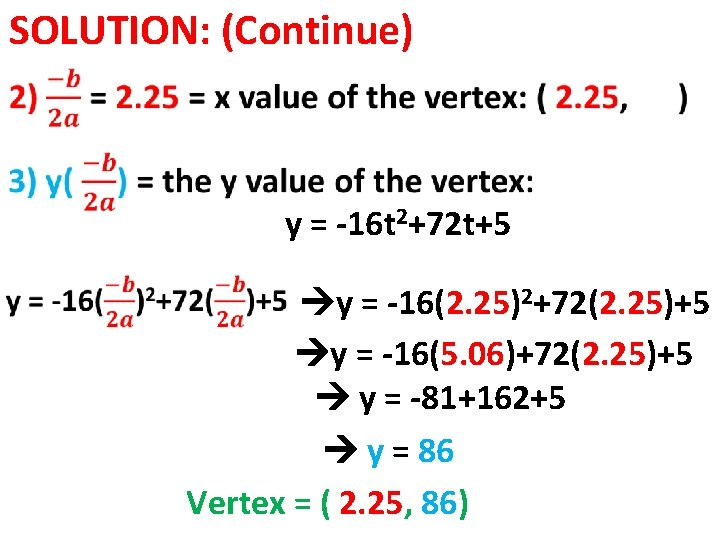SOLUTION: (Continue) y = -16 t 2+72 t+5 y = -16(2. 25)2+72(2. 25)+5 y = -16(5. 06)+72(2. 25)+5 y = -81+162+5 y = 86 Vertex = ( 2. 25, 86)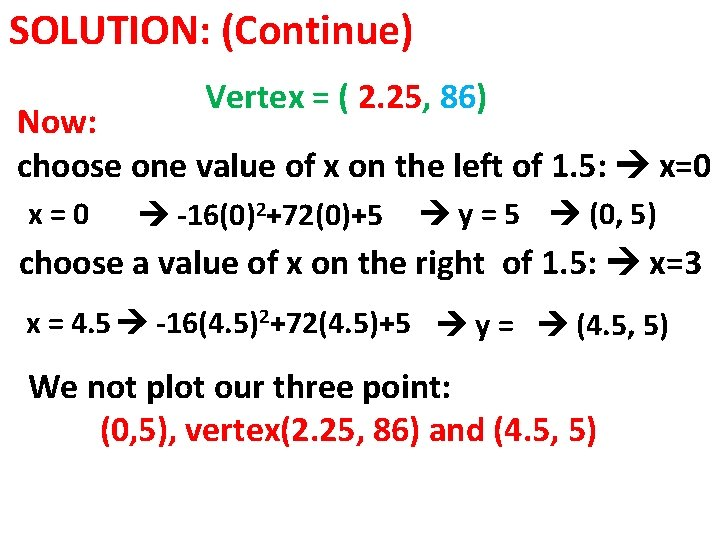SOLUTION: (Continue) Vertex = ( 2. 25, 86) Now: choose one value of x on the left of 1. 5: x=0 -16(0)2+72(0)+5 y = 5 (0, 5) choose a value of x on the right of 1. 5: x=3 x = 4. 5 -16(4. 5)2+72(4. 5)+5 y = (4. 5, 5) We not plot our three point: (0, 5), vertex(2. 25, 86) and (4. 5, 5)SOLUTION: Vertex: ( 2. 25, -86) (0, 5) (4. 5, 5) Domain: (-∞, ∞) Range: (-∞, 86) Maximum : 86 ft.